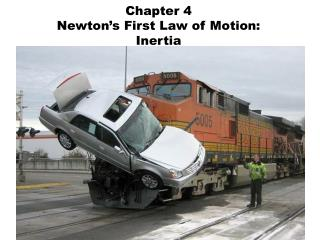Download PresentationChapter 4 Newton’s First Law of Motion: Inertia

# Chapter 4 Newton’s First Law of Motion: Inertia - PowerPoint PPT Presentation

Chapter 4 Newton’s First Law of Motion: Inertia . Force – any push or pull Friction – is the name given to the force that acts between materials that touch as they move past each other .I am the owner, or an agent authorized to act on behalf of the owner, of the copyrighted work described.
Download Presentation## Chapter 4 Newton’s First Law of Motion: Inertia

Download Policy: Content on the Website is provided to you AS IS for your information and personal use and may not be sold / licensed / shared on other websites without getting consent from its author.While downloading, if for some reason you are not able to download a presentation, the publisher may have deleted the file from their server.

- - - - - - - - - - - - - - - - - - - - - - - - - - E N D - - - - - - - - - - - - - - - - - - - - - - - - - -
Presentation Transcript
1. Chapter 4Newton’s First Law of Motion:Inertia

2. Force – any push or pull • Friction – is the name given to the force that acts between materials that touch as they move past each other

3. Galileo showed that a force was not required to keep an object in motion. Two surfaces that come in contact will create some degree of friction, even very smooth surfaces. • If friction were absent, a moving object would need no force to remain in motion

4. Galileo also demonstrated that an object resists changes to its state of motionwhich we call Inertia

5. Inertia Inertia – the tendency of an object to resist a change in its motion • Mass is proportional to inertia

6. Newton’s First Law • Law of Inertia – Every object continues in a state of rest, or of motion in a straight line at constant speed, unless it is compelled to change that state by forces exerted upon it. Restated: • An object at rest (or in motion) tends to stay at rest (or in motion) unless acted on by an outside force

7. Mass vs. Weight Mass - The amount of matter contained in an object • Mass is NOT Volume Example: A bowling ball usually has more mass than a large beach ball • Mass is NOT Weight Example: an astronaut has the same mass in space, but he/she is weightless

8. Mass vs. Weight Continued… • The SI unit of mass is the kilogram (the base unit is the gram) • Weight is mass x gravity • Pounds are the English measurement of weight (mass x gravity) • The Newton is the SI unit of weight

9. The Newton Newton = mass x gravity • Newton = Kilograms x 9.81 m/s2

10. Net Force Net force – the combination of all forces acting on an object Example 1: What is the net force acting on the box? Net Force = 5 Newtons to the right Example 2: What is the net force acting on the box? 10 N 14 N 4 N Net force = 0 Newtons 10 N 5 N

11. Net Force Continued: Normal force (also called the support force) – the upward force that balances the weight of the object. The normal force always acts perpendicular to the plane that the object is resting on. 200 N (Support force in Newtons) 200 N (Weight of box in Newtons)

12. Vector Addition of Forces Example: A box is suspended by a rope The tension in the rope is equal to the weight of the box If two ropes are attached to the same box, then the tension is each rope is half of the weight of the box. 10 N 10 N 5 N 5 N 10 N